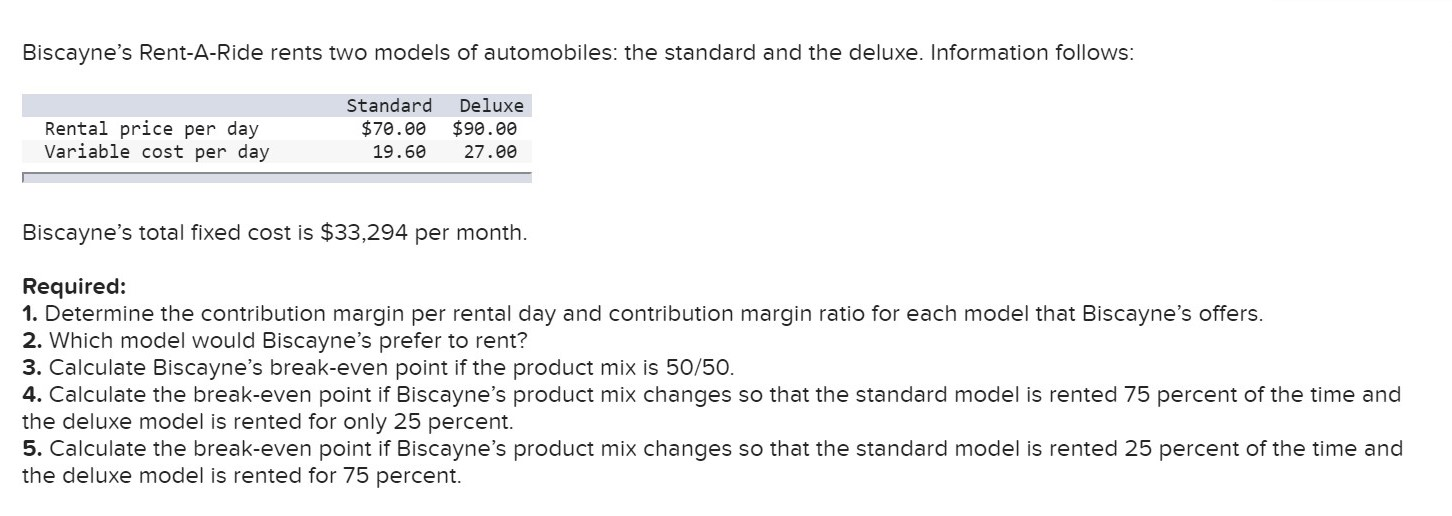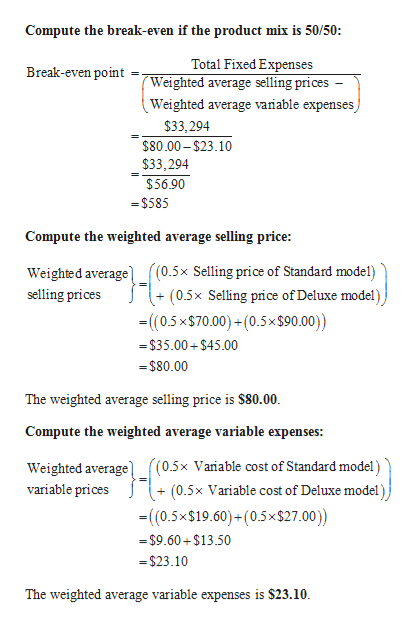# Biscayne's Rent-A-Ride rents two models of automobiles: the standard and the deluxe. Information follows:StandardDeluxeRental price per dayVariable cost per day\$70.00\$90.0019.6027.00Biscayne's total fixed cost is \$33,294 per monthRequired:1. Determine the contribution margin per rental day and contribution margin ratio for each model that Biscayne's offers.2. Which model would Biscayne's prefer to rent?3. Calculate Biscayne's break-even point if the product mix is 50/50.4. Calculate the break-even point if Biscayne's product mix changes so that the standard model is rented 75 percent of the time andthe deluxe model is rented for only 25 percent5. Calculate the break-even point if Biscayne's product mix changes so that the standard model is rented 25 percent of the time andthe deluxe model is rented for 75 percent.

Question
18 viewshelp_outlineImage TranscriptioncloseBiscayne's Rent-A-Ride rents two models of automobiles: the standard and the deluxe. Information follows: Standard Deluxe Rental price per day Variable cost per day \$70.00 \$90.00 19.60 27.00 Biscayne's total fixed cost is \$33,294 per month Required: 1. Determine the contribution margin per rental day and contribution margin ratio for each model that Biscayne's offers. 2. Which model would Biscayne's prefer to rent? 3. Calculate Biscayne's break-even point if the product mix is 50/50. 4. Calculate the break-even point if Biscayne's product mix changes so that the standard model is rented 75 percent of the time and the deluxe model is rented for only 25 percent 5. Calculate the break-even point if Biscayne's product mix changes so that the standard model is rented 25 percent of the time and the deluxe model is rented for 75 percent. fullscreen
check_circle

Step 1

As there are many requirements in this question, we are answering the last three requirements alone.

Step 2

Break-Even Point: The break-even point refers to the point of sales at which the firm neither earns a profit nor suffers a loss. It is also known as the point of sales or sales value at which the firm recovers the entire cost of fixed and variable nature.

Step 3

3)

The break-even if the product mix is 50/50 ...help_outlineImage TranscriptioncloseCompute the break-even if the product mix is 50/50: Total Fixed Expenses Break-even point Weighted average selling prices - Weighted average variable expenses, \$33,294 \$80.00-\$23.10 \$33,294 \$56.90 =\$585 Compute the weighted average selling price ((0.5x Selling price of Standard model) (0.5x Selling price of Deluxe model) (0.5x\$70.00)+(0.5x\$90.00)) Weighted average) selling prices \$35.00+\$45.00 =\$80.00 The weighted average selling price is \$80.00 Compute the weighted average variable expenses: Weighted average (0.5x Variable cost of Deluxe model) ((0.5x Variable cost of Standard model) variable prices (0.5x\$19.60)+(0.5x\$27.00)) \$9.60+\$13.50 \$23.10 The weighted average variable expenses is \$23.10 fullscreen

### Want to see the full answer?

See Solution

#### Want to see this answer and more?

Solutions are written by subject experts who are available 24/7. Questions are typically answered within 1 hour.*

See Solution
*Response times may vary by subject and question.
Tagged in